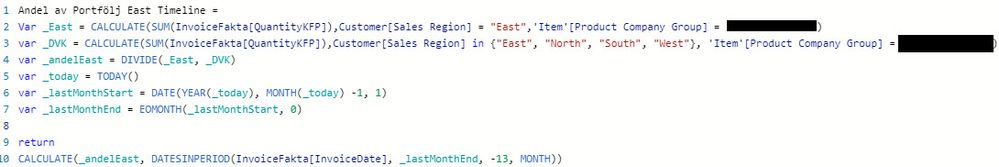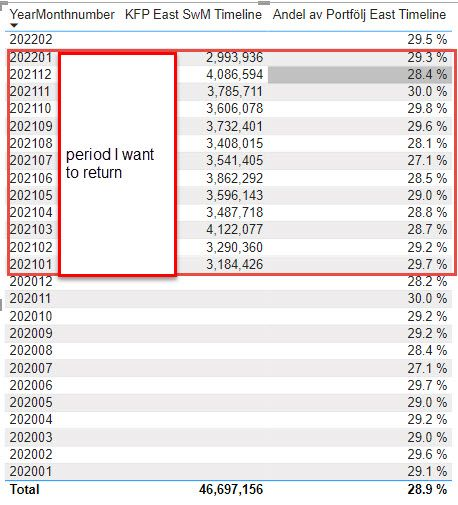cancel
Showing results for
Did you mean:Resolver I

## Datesinperiod is not filtering the period correctly.

Hi,

I am using the same datesinperiod formula to show the last thirteen calender months in two measures: KFP for a region and portfolio share of a region.

KFP for a region uses the following formula, returns the correct values and the correct period.For a portfolio share I am using the formula below. It returns the correct values, however the period is wrong. Please see picture 3 below.The period I expect for both measures is in the red frame below.Could anyone please help me to understand why the same formula is returning different periods and how I can troubleshoot?

1 ACCEPTED SOLUTIONSuper User

Try this.  Make a measure that does just the % calc, no date shifting.   Then you can use that in a measure with DATESINPERIOD and see if that gives us a better result.

``````Andel ev Portfolj East Timeline =
VAR _today = TODAY()
VAR _lastMonthStart = DATE(YEAR(_today), MONTH(_today) -1, 1)
VAR _LastMonthEnd = EOMONTH(lastMonthStart, 0)

RETURN
CALCULATE ( [Andel ev Portfolj East], DATESINPERIOD(InvoiceFakta[InvoideDate], _lastMonthEnd, -13, MONTH ) )``````

The measure called in the CALCULATE will be the one that just calculates the % you are looking for.  Then this measure adjusts the timeframe.

5 REPLIES 5Super User

Try this.  Make a measure that does just the % calc, no date shifting.   Then you can use that in a measure with DATESINPERIOD and see if that gives us a better result.

``````Andel ev Portfolj East Timeline =
VAR _today = TODAY()
VAR _lastMonthStart = DATE(YEAR(_today), MONTH(_today) -1, 1)
VAR _LastMonthEnd = EOMONTH(lastMonthStart, 0)

RETURN
CALCULATE ( [Andel ev Portfolj East], DATESINPERIOD(InvoiceFakta[InvoideDate], _lastMonthEnd, -13, MONTH ) )``````

The measure called in the CALCULATE will be the one that just calculates the % you are looking for.  Then this measure adjusts the timeframe.Resolver I

Thank you, @jdbuchanan71 ! This variant is working. I have otherwise made a workaround and created a column in the date table that sets 1 to the 13 last months and filtered my visual on it.

IsLast13CalenderMonths =
var _today = TODAY()
var _lastMonthStart = DATE(YEAR(_today), MONTH(_today) -1, 1)
var _lastMonthEnd = Format(EOMONTH(_lastMonthStart, 0), "YYYYMM")
var _periodstart = Format(DATE(YEAR(_today), MONTH(_today) -13, 1), "YYYYMM")
return
if('Date'[YearMonthnumber]>= _periodstart && 'Date'[YearMonthnumber]<= _lastMonthEnd, 1,0)Super User

What is it returning after you changed it?Resolver I

@jdbuchanan71  Thank you for the tip! Unfortunately it is still returning the wrong period.

Is datesinperiod is supposed to filter on the dates period? Can it be a bugg?Super User

I think you are getting into trouble using CALCULATE on a variable.  Try the last line of your second measure like this.

``````RETURN
CALCULATE ( DIVIDE ( _East, _DVK), DATESINPERIOD(InvoiceFakta[InvoideDate], _lastMonthEnd, -13, MONTH ) )``````